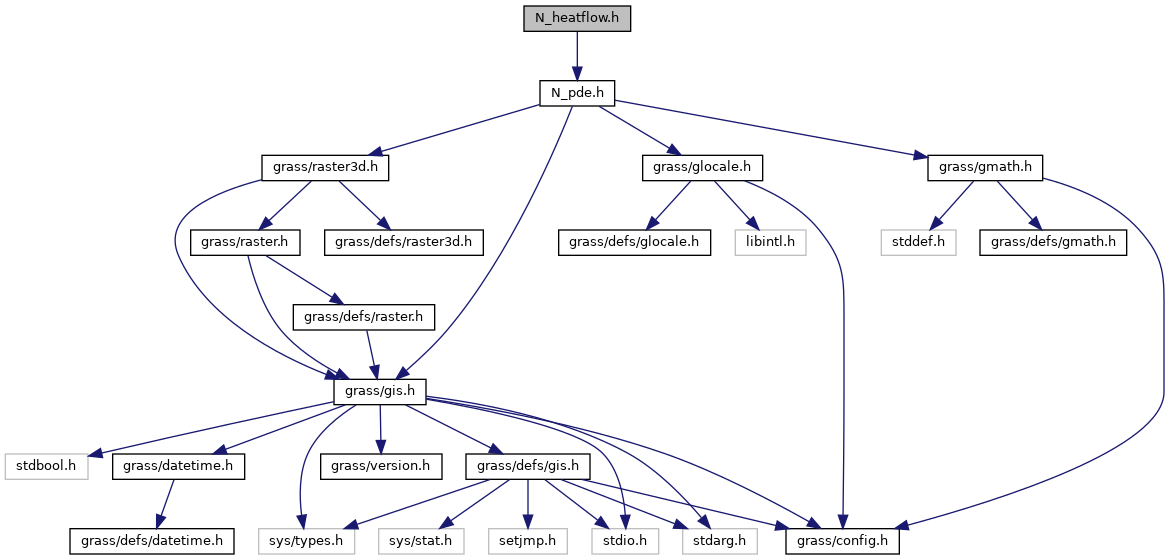GRASS GIS 7 Programmer's Manual  7.9.dev(2021)-e5379bbd7
N_heatflow.h File Reference
`#include "N_pde.h"`
Include dependency graph for N_heatflow.h:Go to the source code of this file.

## Data Structures

struct  N_heatflow_data3d

struct  N_heatflow_data2d

## Functions

N_data_starN_callback_heatflow_3d (void *heatdata, N_geom_data *geom, int depth, int row, int col)

N_data_starN_callback_heatflow_2d (void *heatdata, N_geom_data *geom, int row, int col)

N_heatflow_data3dN_alloc_heatflow_data3d (int depths, int rows, int cols)

N_heatflow_data2dN_alloc_heatflow_data2d (int rows, int cols)

void N_free_heatflow_data3d (N_heatflow_data3d *data)

void N_free_heatflow_data2d (N_heatflow_data2d *data)

## ◆ N_alloc_heatflow_data2d()

 N_heatflow_data2d* N_alloc_heatflow_data2d ( int rows, int cols )

## ◆ N_alloc_heatflow_data3d()

 N_heatflow_data3d* N_alloc_heatflow_data3d ( int depths, int rows, int cols )

## ◆ N_callback_heatflow_2d()

 N_data_star* N_callback_heatflow_2d ( void * heatdata, N_geom_data * geom, int row, int col )

## ◆ N_callback_heatflow_3d()

 N_data_star* N_callback_heatflow_3d ( void * heatdata, N_geom_data * geom, int depth, int row, int col )

## ◆ N_free_heatflow_data2d()

 void N_free_heatflow_data2d ( N_heatflow_data2d * data )

## ◆ N_free_heatflow_data3d()

 void N_free_heatflow_data3d ( N_heatflow_data3d * data )